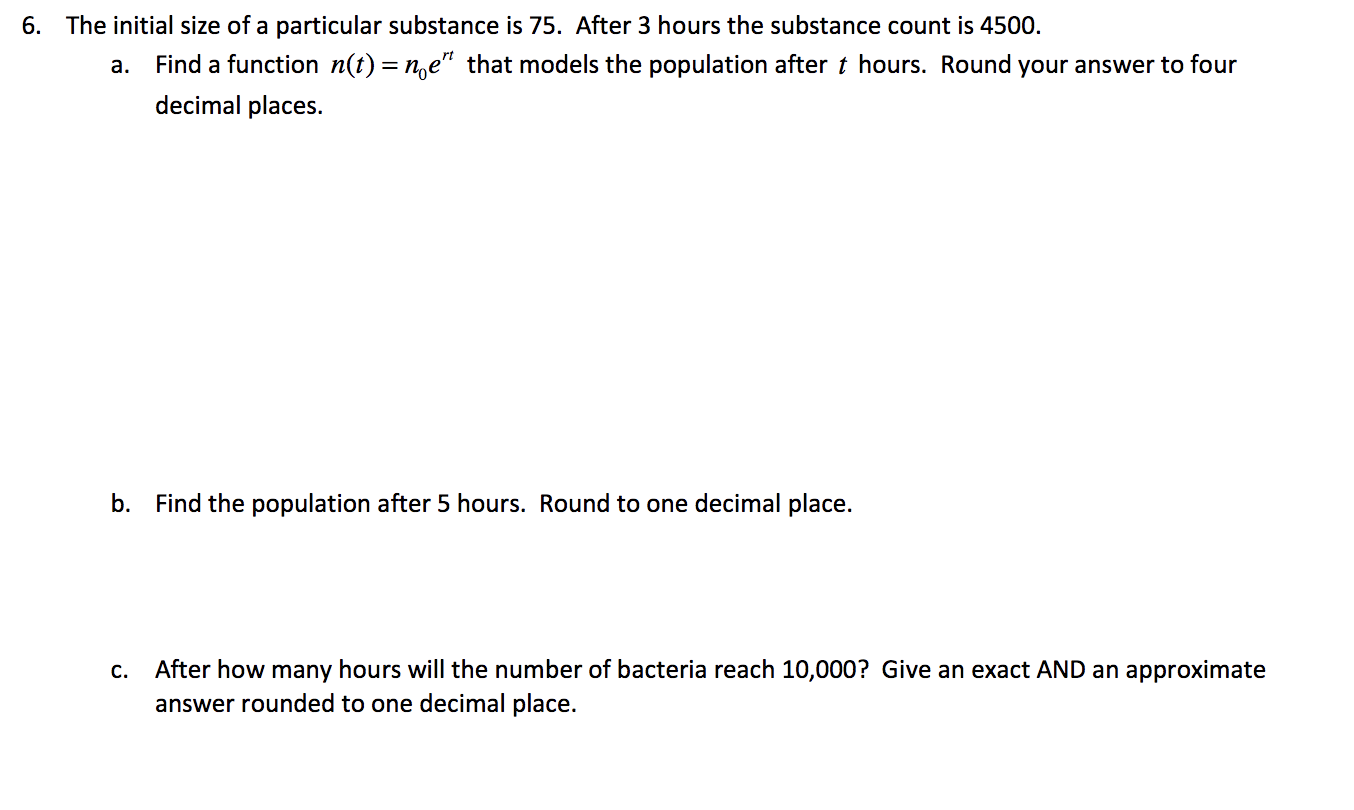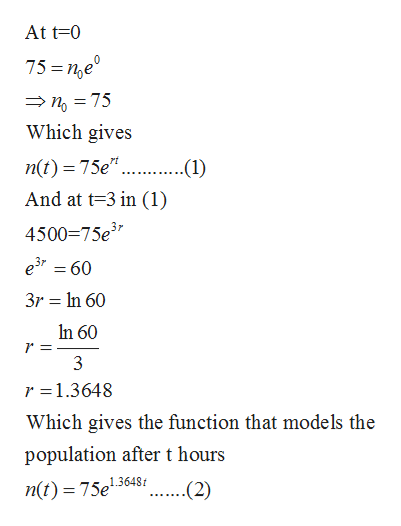# The initial size of a particular substance is 75. After 3 hours the substance count is 4500.6.Find a function n(t) = ne" that models the population after t hours. Round your answer to fourа.decimal places.Find the population after 5 hours. Round to one decimal placeb.After how many hours will the number of bacteria reach 10,000? Give an exact AND an approximateanswer rounded to one decimal place.С.

Question
3 viewshelp_outlineImage TranscriptioncloseThe initial size of a particular substance is 75. After 3 hours the substance count is 4500. 6. Find a function n(t) = ne" that models the population after t hours. Round your answer to four а. decimal places. Find the population after 5 hours. Round to one decimal place b. After how many hours will the number of bacteria reach 10,000? Give an exact AND an approximate answer rounded to one decimal place. С. fullscreen
check_circle

Step 1

Given function which models the population after t hours is

Step 2

(a)Given that initial size of a particular substance is 75. After 3 hours the substance is count is 4500. So,help_outlineImage TranscriptioncloseAt t 0 75 ne ng75 Which gives n(t) 75e ...(1 And at t-3 in (1) 4500 75e3 e3 60 3r In 60 In 60 3 r 1.3648 Which gives the function that mode ls the population after t hours (2) n(t) = 75e136481 fullscreen
Step 3

(b)Population aft...

### Want to see the full answer?

See Solution

#### Want to see this answer and more?

Solutions are written by subject experts who are available 24/7. Questions are typically answered within 1 hour.*

See Solution
*Response times may vary by subject and question.
Tagged in

### Other